Next: Point Sources Up: Axisymmetric Incompressible Inviscid Flow Previous: Axisymmetric Irrotational Flow in

# Uniform Flow

Consider a uniform steady stream of velocity. Consider the flux (in the minus-direction) across a plane circle of radius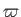that lies in the-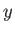plane, and whose center coincides with the-axis. From the definition of the Stokes stream function (see Section 7.3), we have, or(7.25)

When expressed in terms of spherical coordinates, the previous expression yields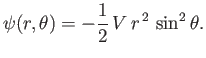(7.26)

Of course, uniform flow is irrotational [this is clear from a comparison of Equations (7.10) and (7.25)], so we can also represent the flow pattern in terms of a velocity potential: that is (see Section 5.4),(7.27)

or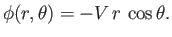(7.28)

It follows, from the previous analysis, that the velocity field of a uniform stream, running parallel to the-axis, can either be written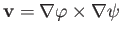, with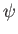specified by Equations (7.25)-(7.26), or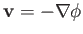, withspecified by Equations (7.27)-(7.28).Next: Point Sources Up: Axisymmetric Incompressible Inviscid Flow Previous: Axisymmetric Irrotational Flow in
Richard Fitzpatrick 2016-03-31